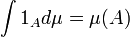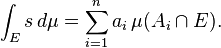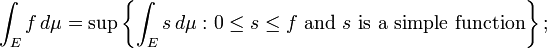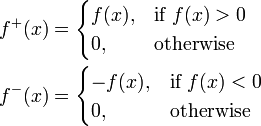# LEBESGUE INTEGRAL

The Riemann integral is not defined for a wide range of functions and situations of importance in applications (and of interest in theory). For example, the Riemann integral can easily integrate density to find the mass of a steel beam, but cannot accommodate a steel ball resting on it. This motivates other definitions, under which a broader assortment of functions are integrable (Rudin 1987). The Lebesgue integral, in particular, achieves great flexibility by directing attention to the weights in the weighted sum.

The definition of the Lebesgue integral thus begins with a measure, μ. In the simplest case, the Lebesgue measure μ(A) of an interval A = [a,b] is its width, ba, so that the Lebesgue integral agrees with the (proper) Riemann integral when both exist. In more complicated cases, the sets being measured can be highly fragmented, with no continuity and no resemblance to intervals.

To exploit this flexibility, Lebesgue integrals reverse the approach to the weighted sum. As Folland (1984, p. 56) puts it, “To compute the Riemann integral of f, one partitions the domain [a,b] into subintervals”, while in the Lebesgue integral, “one is in effect partitioning the range of f“.

One common approach first defines the integral of the indicator function of a measurable set A by:.

This extends by linearity to a measurable simple function s, which attains only a finite number, n, of distinct non-negative values:(where the image of Ai under the simple function s is the constant value ai). Thus if E is a measurable set one definesThen for any non-negative measurable function f one definesthat is, the integral of f is set to be the supremum of all the integrals of simple functions that are less than or equal to f. A general measurable function f, is split into its positive and negative values by defining0 \\
0, & \text{otherwise}
\end{cases} \\
f^-(x) &{}= \begin{cases}
-f(x), & \text{if } f(x)

Finally, f is Lebesgue integrable if

<img src="http://upload.wikimedia.org/math/9/2/3/923f8b74b92d6699f4c3bbefb00496b0.png&quot; alt="\int_E |f| \, d\mu

and then the integral is defined byWhen the measure space on which the functions are defined is also a locally compact topological space (as is the case with the real numbers R), measures compatible with the topology in a suitable sense (Radon measures, of which the Lebesgue measure is an example) and integral with respect to them can be defined differently, starting from the integrals of continuous functions with compact support. More precisely, the compactly supported functions form a vector space that carries a natural topology, and a (Radon) measure can be defined as any continuous linear functional on this space; the value of a measure at a compactly supported function is then also by definition the integral of the function. One then proceeds to expand the measure (the integral) to more general functions by continuity, and defines the measure of a set as the integral of its indicator function. This is the approach taken by Bourbaki (2004) and a certain number of other authors. For details see Radon measures.Welcome to my blog. My name is Nico. Admin of this blog. I am a student majoring in mathematics who dreams of becoming a professor of mathematics. I live in Kwadungan, Ngawi, East Java. Hopefully in all the posts I can make a good learning material to the intellectual life of the nation. After the read, leave a comment. I always accept criticism suggestion to build a better me again .. Thanks for visiting .. : mrgreen:

Posted on August 30, 2011, in education and tagged , , , , , , , . Bookmark the permalink. 3 Comments.

1.Medicine Of Herbal

wew….
gmn cara masukin rumus2 gt mas???

2.Putri

Satukan tangan, satukan hati

Itulah indahnya silaturahmi

•NICO VENGEANCE